# Test: Definite Integral (Competition Level) - 2

## 30 Questions MCQ Test Mathematics (Maths) Class 12 | Test: Definite Integral (Competition Level) - 2

Description
This mock test of Test: Definite Integral (Competition Level) - 2 for JEE helps you for every JEE entrance exam. This contains 30 Multiple Choice Questions for JEE Test: Definite Integral (Competition Level) - 2 (mcq) to study with solutions a complete question bank. The solved questions answers in this Test: Definite Integral (Competition Level) - 2 quiz give you a good mix of easy questions and tough questions. JEE students definitely take this Test: Definite Integral (Competition Level) - 2 exercise for a better result in the exam. You can find other Test: Definite Integral (Competition Level) - 2 extra questions, long questions & short questions for JEE on EduRev as well by searching above.
QUESTION: 1

### If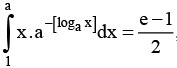, where a >1 and [.] denotes the greatest integer function, then the value of a2 is

Solution: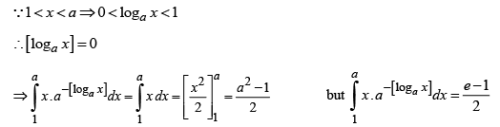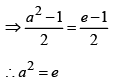QUESTION: 2

### Number of positive continuous functions f (x ) defined in [0,1] for which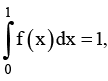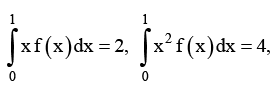is

Solution:

Multiplying these integrals by 4, -4,1 and adding we get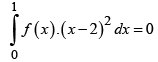Hence, there does not exist any function satisfying these conditions.

QUESTION: 3

### For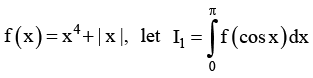and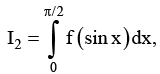has the  value equal to

Solution: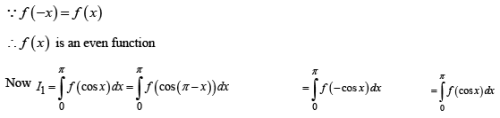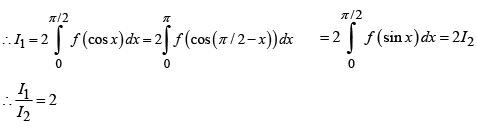QUESTION: 4

If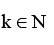and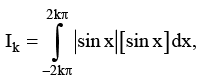where [.] denotes the greatest integer function,  then the value of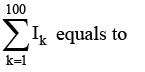equals to

Solution: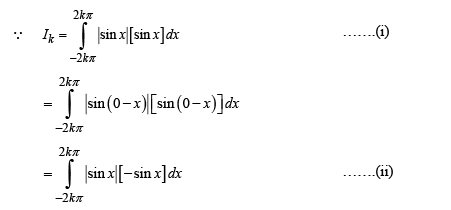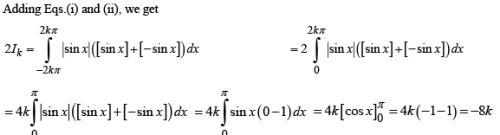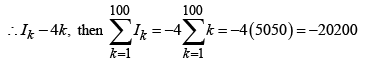QUESTION: 5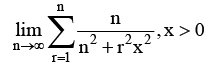is equal to

Solution: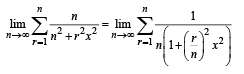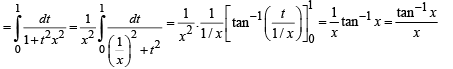QUESTION: 6

If f is continuous function and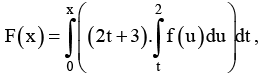then the value  of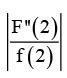is

Solution: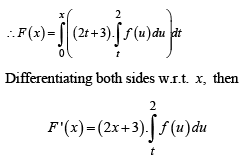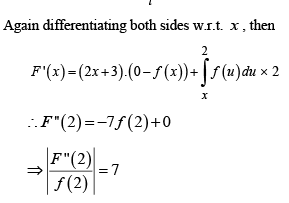QUESTION: 7

Let a >0 and f (x ) is monotonic increasing such that f ( 0) = 0 and f ( a ) = b, then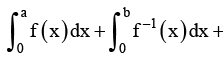is equal to

Solution: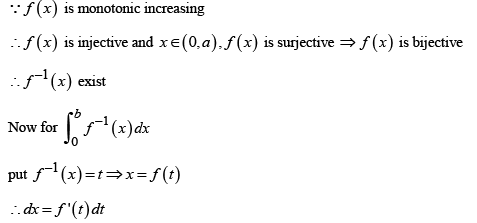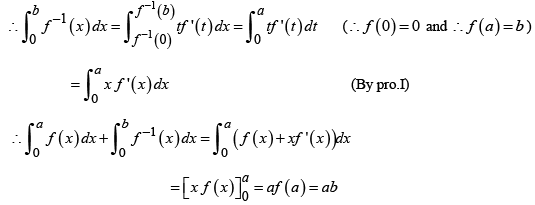QUESTION: 8

If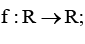f ( x ) = x+ sin x, then the value of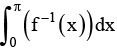is equal to

Solution: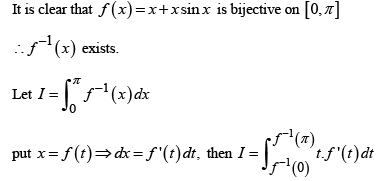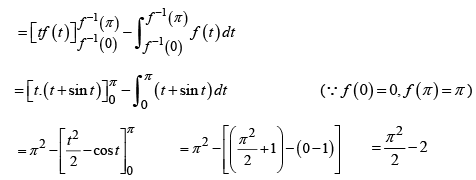QUESTION: 9

If f ( x ) = sin x+ cos x and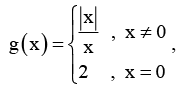, then the value  of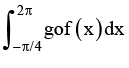is   equal to

Solution: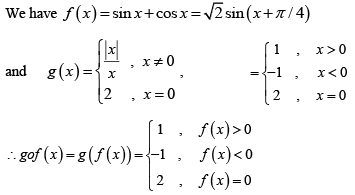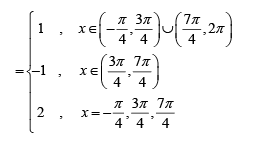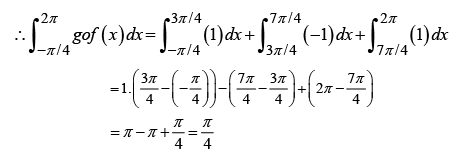QUESTION: 10

Let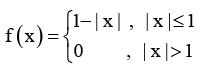and g ( x ) = f ( x - 1) + f ( x + 1) for all x ÎR , then the  of value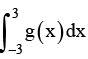is

Solution: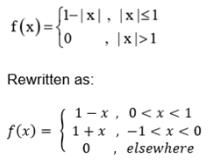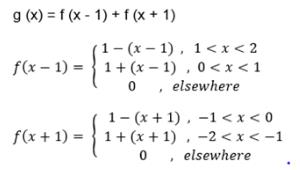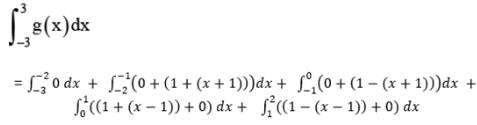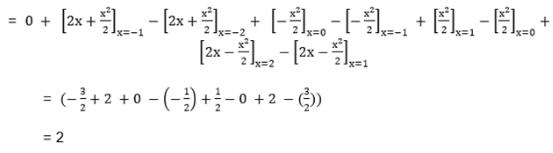QUESTION: 11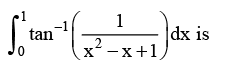Solution: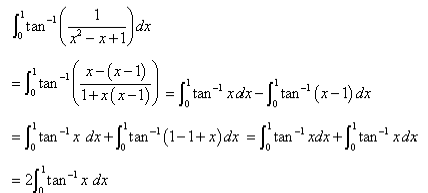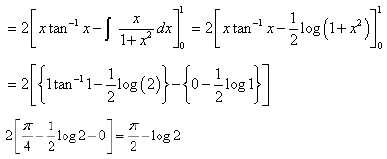QUESTION: 12

Let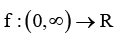and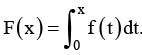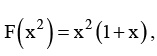then f ( 4 ) equals

Solution: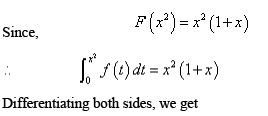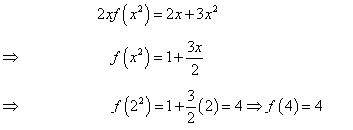QUESTION: 13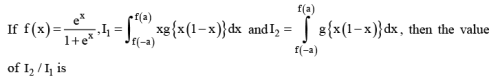Solution:
QUESTION: 14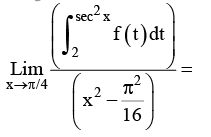Solution:
QUESTION: 15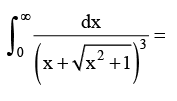Solution:
QUESTION: 16

If the value of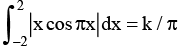then the value of k is

Solution: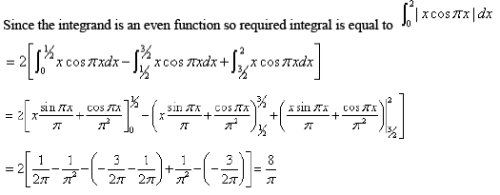QUESTION: 17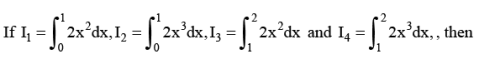Solution: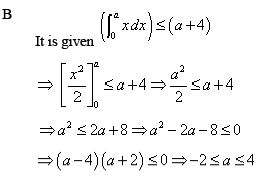QUESTION: 18

if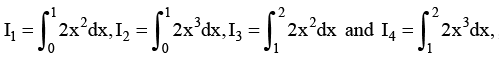then

Solution:
QUESTION: 19

If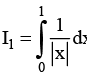and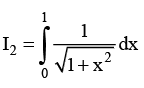then

Solution:
QUESTION: 20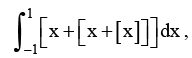where [.] denote greatest integer function, is equal to

Solution: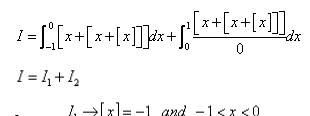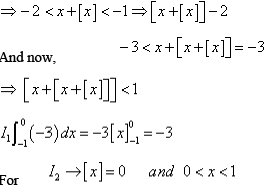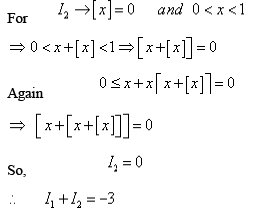QUESTION: 21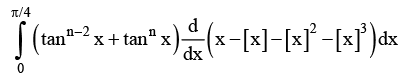,where denotes greatest integer function is given by

Solution: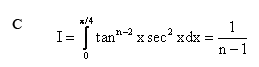QUESTION: 22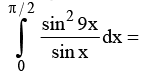Solution:
QUESTION: 23

Let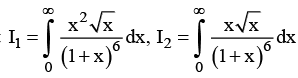then

Solution:
QUESTION: 24

If [.] denotes G.I.F,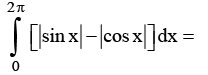Solution: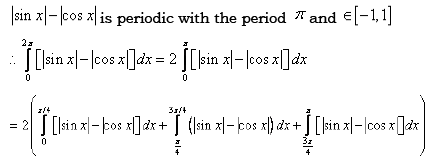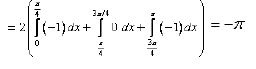QUESTION: 25

The value of constant a >0 such that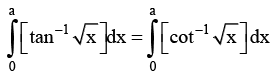where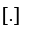denotes G.I.F is ___

Solution: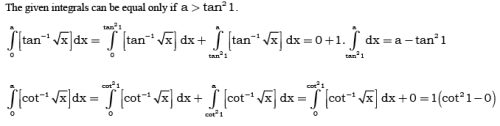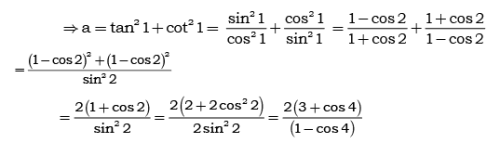QUESTION: 26

A function f is defined by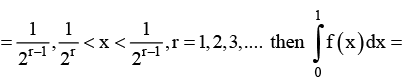Solution: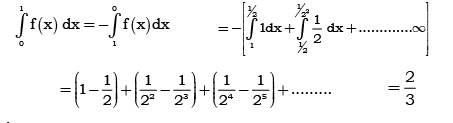QUESTION: 27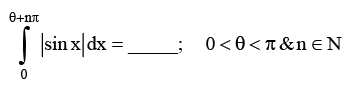Solution:
QUESTION: 28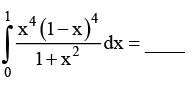Solution:
QUESTION: 29

If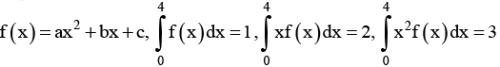Then in the interval  (0,4); f(x)=0 has

Solution: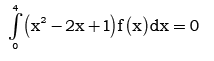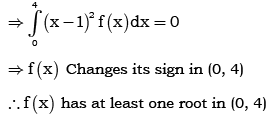QUESTION: 30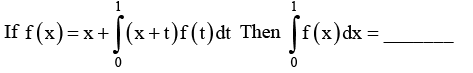Solution: